﻿ 信道天文学(简介)：天文信号的信道模式处理

# 信道天文学(简介)：天文信号的信道模式处理Channel Astronomy (Brief Introduction): Astronomical Signal Channel Processing Mode

Abstract: Based on the modern communication model, this paper deals with the channel interaction of as-tronomical signals, especially the signals from distant extragalactic galaxies and supernovae, and draws different conclusions from current astronomy, which are more consistent with the observa-tions of astronomical phenomena such as cosmic red shift and supernova signals. The conclusion of the channel mode of processing of astronomical signals is that galaxies do not need to retreat, and the universe does not have a big explosion. The cosmic red shift is only caused by the propagation of light wave signals in space channels.

1. 天文信号处理模式发展过程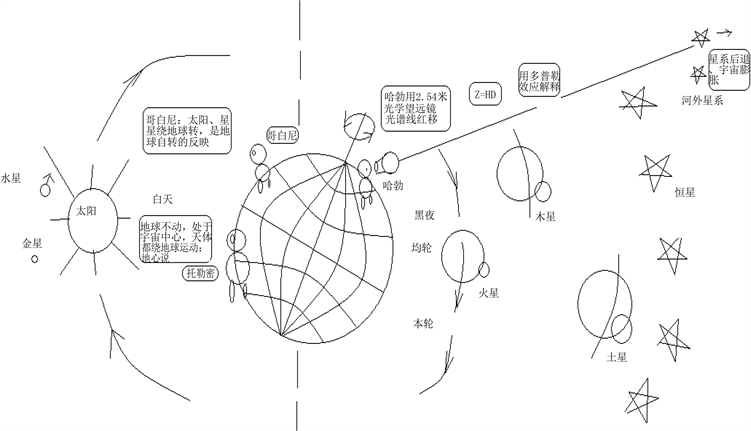Figure 1. Ptolemy (geocentric theory), Copernicus (heliocentric theory), Hubble (Big Bang) stood on the earth, observing and interpreting astronomical information

${V}_{f}={H}_{C}×D$ (1)

2. 信道天文学：天文信号的信道模式处理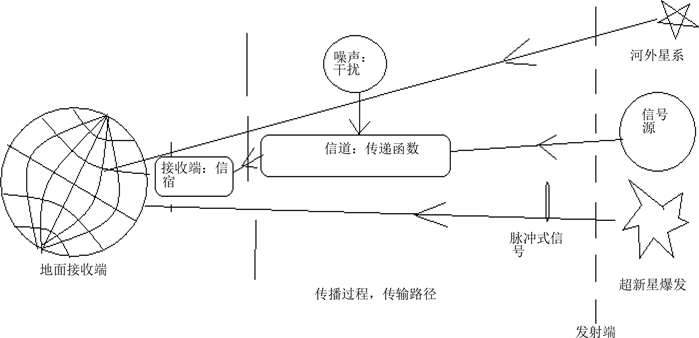Figure 2. Processing astronomical signals using modern communication models

$Y\left(x,t\right)=H\left(x,t\right)*s\left(t\right)+n\left(t\right)$ (2)

$s\left(t\right)=\frac{y\left(x,t\right)-n\left(t\right)}{H\left(x,t\right)}$ (3)

3. 宇宙红移的信道模式处理

$s\left(t\right)=A\mathrm{sin}\varpi t$ (4)

$I\left(x\right)=I\left({x}_{0}\right){e}^{-2\alpha x}$ (5)

$A\left(x\right)=A\left({x}_{0}\right){e}^{-\alpha x}$ (6)

${f}_{o}={f}_{s}{e}^{-{\alpha }_{f}x}$${\lambda }_{o}={\lambda }_{s}{e}^{-{\alpha }_{f}x}$ (7)

$Z=\frac{{\lambda }_{o}-{\lambda }_{s}}{{\lambda }_{s}}$ (8)

$Z=\frac{{\lambda }_{o}-{\lambda }_{s}}{{\lambda }_{s}}={e}^{{\alpha }_{f}x}-1$ (9)

$Z={e}^{{\alpha }_{f}x}-1\approx {\alpha }_{f}x$ (10)

1 Mpc = 106 pc = 3.2616 * 106 l.y. = 3.2616 * 106 * 0.94607 * 1016 m = 3.08568 * 1022 m；

H1 m = 0.8 * 10−26/m (11)

$Z=\frac{{\lambda }_{o}-{\lambda }_{i}}{{\lambda }_{i}}\approx \frac{{f}_{i}-{f}_{o}}{{f}_{i}}$ (12)

$\nabla f={f}_{i}-{f}_{o}\approx {f}_{i}*Z=Z*D*{f}_{i}$ (13)

$\Delta \theta =2\text{π}*\Delta t*\Delta f=2\text{π}*\Delta t*Z*{f}_{i}=2\text{π}*\Delta t*HD*{f}_{i}$ (14)

$\Delta \theta$ = 360˚ * 86400 s * 0.8 * 10−26/m * 2 * 104 m * 1015 Hz/s = 5˚(15)

4. 超新星信号的信道模式处理

${a}_{n}\frac{{d}^{n}y\left(t\right)}{d{t}^{n}}+\cdots +{a}_{0}y={b}_{m}\frac{{d}^{m}x}{d{t}^{m}}+\cdots +{b}_{0}x$ (16)

$\delta \left(t\right)=\left[\begin{array}{l}\infty ,t=0\\ 0,t\ne 0\end{array}\text{\hspace{0.17em}}与\text{\hspace{0.17em}}\underset{-\infty }{\overset{+\infty }{\int }}\delta \left(t\right)\text{d}t=A$ (17)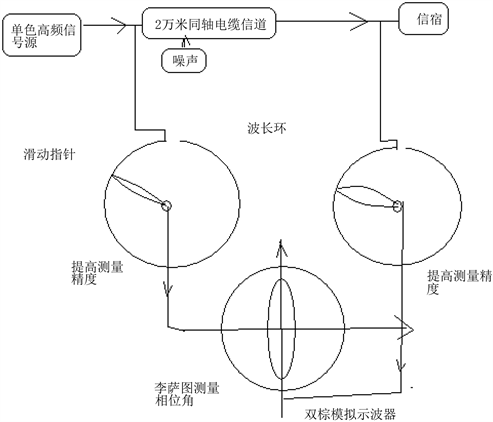Figure 3. Experimental diagram for measuring frequency attenuation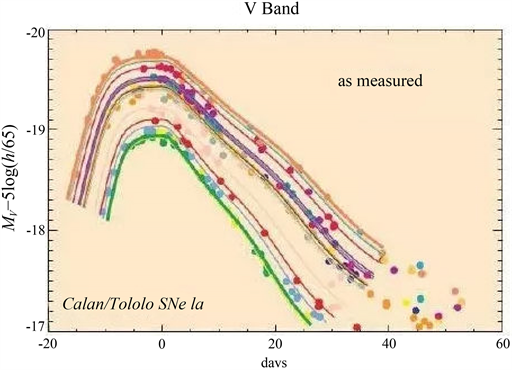Figure 4. Supernova signal observations (copy reference)

${a}_{2}\frac{\text{d}{S}_{o}^{2}}{\text{d}{t}^{2}}+{a}_{1}\frac{\text{d}{S}_{o}\left(t\right)}{\text{d}t}+{a}_{0}{S}_{o}\left(t\right)={b}_{0}{S}_{i}\left(t\right)$ (18)

$H\left(S\right)=\frac{{b}_{0}}{{a}_{2}{s}^{2}+{a}_{1}s+{a}_{0}}$ (19)

1) 临界阻尼状态响应：

${S}_{o}\left(t\right)=\frac{{b}_{0}}{{a}_{2}}*t*{e}^{-\sqrt{\frac{{a}_{0}}{{a}_{2}}}*t}$ (20)

2) 过阻尼状态

${S}_{o}\left(t\right)=\frac{{\varpi }_{0}k}{2\sqrt{{\xi }^{2}-1}}\left[{\text{e}}^{-\left(\xi +\sqrt{{\xi }^{2}-1}\right){\varpi }_{0}t}-{\text{e}}^{{}^{-\left(\xi -\sqrt{{\xi }^{2}-1}\right){\varpi }_{0}t}}\right]$ (21)

3) 欠阻尼状态

${S}_{o}\left(t\right)=\frac{{\varpi }_{0}k}{2\sqrt{1-{\xi }^{2}}}\left[{\text{e}}^{-\xi {\varpi }_{0}t}\mathrm{sin}\left(\sqrt{1-{\xi }^{2}}{\varpi }_{0}t\right)\right]$ (22)

${S}_{o}\left(t\right)=0.41t*{\text{e}}^{-0.05977t}$ (23)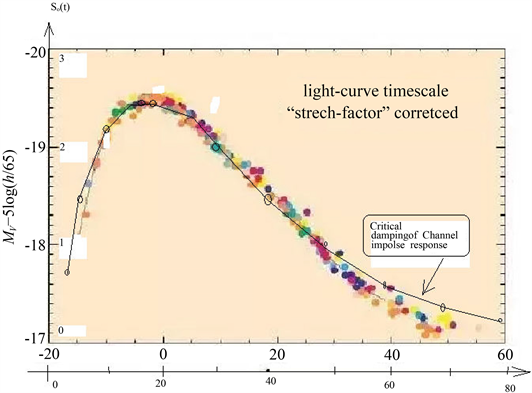Figure 5. Fitting with the second order channel critical damping impulse response function

2011年诺贝尔获奖者成果：发现超新星亮度比预期的低、红移比预期的大

4.1. 亮度比预期低的信道解释

$M-m=-2.5\mathrm{log}\left(\frac{{F}_{10}}{{F}_{D}}\right)=5-5\mathrm{log}d\left(pc\right)$ (24)

${S}_{o}\left(t\right)=\frac{{b}_{0}}{{a}_{2}}*t*{e}^{-\sqrt{\frac{{a}_{0}}{{a}_{2}}}*t}$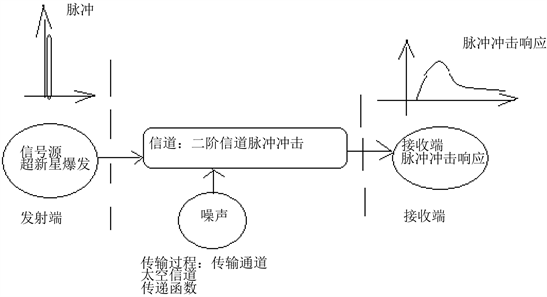Figure 6. Impact response of pulse function to channel

4.2. 红移比预期的大

${\lambda }_{o}={\lambda }_{s}{e}^{{\alpha }_{f}d}$

$Z=\frac{{\lambda }_{o}-{\lambda }_{s}}{{\lambda }_{s}}={e}^{{\alpha }_{f}d}-1\approx {\alpha }_{f}d$

${S}_{o}\left(t\right)\frac{{b}_{0}}{{a}_{2}}*t*{e}^{-\sqrt{\frac{{a}_{0}}{{a}_{2}}}*t}$

5. 信道对波的弥散作用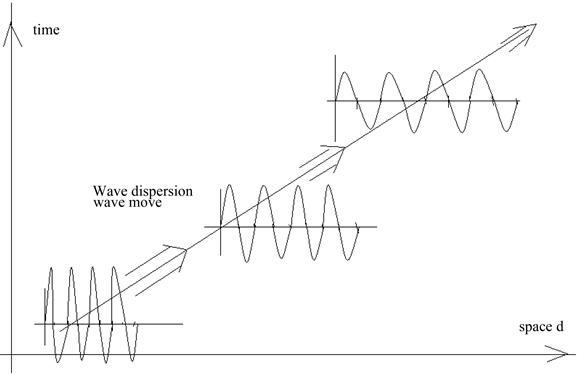Figure 7. Dispersion diagram of wave pulses by channel

6. 结论

 伽利略. 关于托勒枚和哥白尼两大世界体系的对话[M]. 上海: 上海人民出版社, 1974: 242-243.

 哥白尼. 天体运行论[M]. 叶式辉, 译. 武汉: 武汉出版社, 1992: 15-16.

 伊萨克∙牛顿. 自然哲学之数学原理: 宇宙体系[M]. 王克迪, 译. 武汉: 武汉出版社, 1992.

 戴文赛. 太阳系演化学[M]. 北京: 科学出版社, 1980.

 李艳平, 申先甲. 物理学史教程[M]. 北京: 科学出版社, 2003: 102.

 凯德洛夫, 等, 主编. 物理学的方法论原理[M]. 柳树滋, 等, 译. 北京: 知识出版社, 1990: 447.

 关士续, 等. 自然辩证法概论[M]. 北京: 高等教育出版社, 1991.

 Yuksel, H., Kistler, M.D., Beacom, J.F. and Hopkins, A.M. (2008) Revealing the High-Redshift Star Formation Rate with Gamma-Ray Bursts. The Astrophysical Journal, 683, L5.
https://doi.org/10.1086/591449

 Wang, F.Y. and Dai, Z.G. (2009) High Red-Shift Star Formation Rate up to Z~8.3 Derived from Gamma-Ray Bursts and Influence of Background Cosmology. Monthly Notices of Royal Astronomical Society, 400, L10.

 Dado, S. and Dar, A. (2013) Long Gamma-Ray Bursts Trace the Star Formation History. The Astrophysical Journal, 785, 70.

 Wang, F.Y. (2013) The High-Redshift Star Formation Rate Derived from Gamma-Ray Bursts: Possible Origin and Cosmic Reionization. Astronomy & Astrophysics, 556, A90.

 Hopkins, A.M. and Bencom, J.F. (2006) On the Normalization of the Cosmic Star Formation History. The Astrophysical Journal, 651, 142-154.

 Sokolov, V.V. (2011) The Gamma-Ray Bursts and Core-Collapse Supernovae and Global Star Forming Rate at Large Red-Shifts. Bulletin of the Astronomical Society of India, 1-15.

 Perlmutter, S., et al. (1999) Measurements of Ω and Λ from 42 High-Redshift Supernovae. The Astrophysical Journal, 517, 565-586.

 Dong, S., et al. (2016) ASASSN-15lh: A Highly Super-Luminous Supernova. Science, 351, 257-260.
https://doi.org/10.1126/science.aac9613

 Rhee, M.Y. (2002) CDMA Cellular Mobile Communications and Network Security. Prentice Hall, Upper Saddle River, NJ.

 Feher, K. (1995) Wireless Digital Communication. Prentice Hall, Upper Saddle River, NJ.

 GSM Recommendation 03.01, ETS300 521-1994, 1994, 1.

 Communications Week, 1993. 9.

 Rappaport, T.S. (1996) Wireless Communications Principles & Practice. Prentice Hall, Upper Saddle River, NJ.

 郭梯云, 邬国杨, 李建东. 移动通信[M]. 西安: 西安电子科技大学出版社, 2001.

 李兆玉, 何维, 戴翠琴. 移动通信[M]. 北京: 电子工业出版社, 2017.

 陈显治, 等, 编著. 现代通信技术[M]. 北京: 电子工业出版社, 2004.

 赵洪波, 卜益民, 陈风娟, 编著. 现代通信技术概论[M]. 北京: 北京邮电大学出版社, 2003.

 张传福, 彭灿, 胡熬, 刘晓甲, 卢辉斌, 编著. CDAM移动通信网络规划设计与优化[M]. 北京: 人民邮电出版社, 2006.

 苏华鸿, 孙儒石, 等, 编著, 蜂窝移动通信射频工程[M]. 北京: 人民邮电出版社, 2005.

 冯玉珉, 编著. 通信系统原理[M]. 北京: 清华大学出版社, 2003.

 Saml Tabbans. 无线移动通信网络[M]. 李新付, 等, 译. 北京: 电子工业出版社, 2001.

 孙宇彤, 等, 编著. CDMA空中接口技术[M]. 北京: 人民邮电出版社, 2004.

 王家礼, 等. 电磁场与电磁波[M]. 西安: 西安电子科技大学出版社, 2000.

 陈宇, 陈寿元. 天文信息的处理模式研究[J]. 天线学报, 2018, 7(4): 25-34.
http://www.hanspub.org/journal/ja

 陈宇, 陈寿元. 基于现代通信模型的遥远星系光色变化研究[J]. 山东师范大学学报(自然科学版), 2019, 34(1): 61-69.

 陈寿元. 频率衰减: 哈勃红移——陈寿元效应[J]. 科技信息, 2017(11): 50.

 孙学康, 张金菊, 光纤通信技术[M]. 北京: 北京邮电大学出版社, 2001.

Top